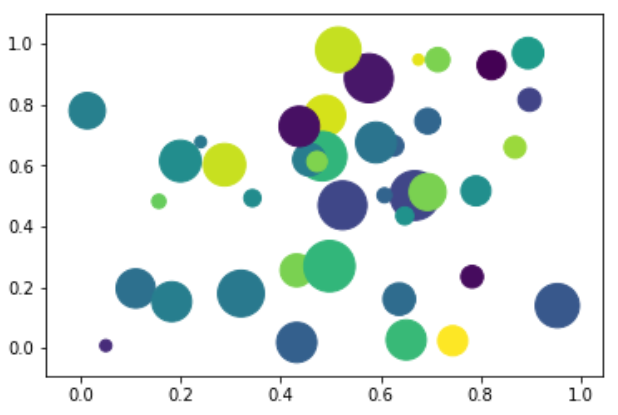# Python - Bubble Charts

Bubble charts display data as a cluster of circles. The required data to create bubble chart needs to have the xy coordinates, size of the bubble and the colour of the bubbles. The colours can be supplied by the library itself.

## Drawing a Bubble Chart

Bubble chart can be created using the DataFrame.plot.scatter() methods.

```import matplotlib.pyplot as plt
import numpy as np

# create data
x = np.random.rand(40)
y = np.random.rand(40)
z = np.random.rand(40)
colors = np.random.rand(40)
# use the scatter function
plt.scatter(x, y, s=z*1000,c=colors)
plt.show()
```

Its output is as follows −## Useful Video Courses

Video

#### Python Online Training

187 Lectures 17.5 hours

Video

#### Python Essentials Online Training

55 Lectures 8 hours

Video

#### Learn Python Programming in 100 Easy Steps

136 Lectures 11 hours

Video

#### Python with Data Science

Best Seller

75 Lectures 13 hours

Video

#### Python 3 from scratch to become a developer in demand

Best Seller

70 Lectures 8.5 hours

Video

#### Python Data Science basics with Numpy, Pandas and Matplotlib

Most Popular

63 Lectures 6 hours